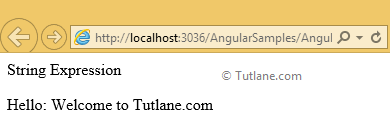String Expression in AngularJS with Examples

Here we will learn what are the string expressions in angularjs and how to use string expressions in angularjs with examples.

String Expressions in AngularJs

The string expression in angularjs is a unit of code to perform operations on string values. We will see how to use string expressions in angularjs with example

Example of String Expressions in AngularJS

<script type="text/javascript">

var app = angular.module('expressionApp', [])

app.controller("expressionController", function (\$scope) {

\$scope.expression1 = "Welcome to ";

\$scope.expression2 = "Tutlane.com"

});

</script>

Write the following code under starting of body tag <body> for string expression in angularjs like as shown below.

<body ng-app="expressionApp">

<form id="form1">

<div ng-controller="expressionController">

<div>String  Expression</div>

<p>Hello: {{expression1+" "+expression2}}</p>

</div>

</form>

</body>

Following is the full example code for string expressions in angularjs with live demo as shown below

<html xmlns="http://www.w3.org/1999/xhtml">

<title>String Expressions of AngularJs</title>

<script type="text/javascript">

var app = angular.module('expressionApp', [])

app.controller("expressionController", function (\$scope) {

\$scope.expression1 = "Welcome to ";

\$scope.expression2 = "Tutlane.com"

});

</script>

<body ng-app="expressionApp">

<form id="form1">

<div ng-controller="expressionController">

<div>String  Expression</div>

<p>Hello: {{expression1+" "+expression2}}</p>

</div>

</form>

</body>

</html>

Now run the above code of string expressions in angularjs example and check the output that will be like as shown below

Output of AngularJS String Expressions

Following is the result of angularjs string expressions example.